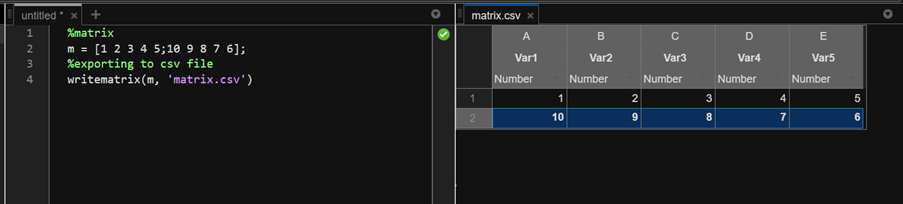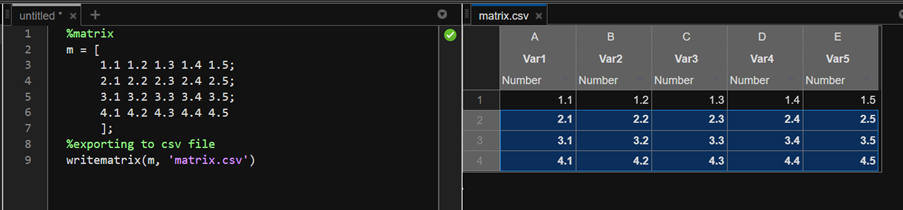GeeksforGeeks App
Open AppBrowser
Continue

# How To Export a Matrix as a CSV File in MATLAB?

A CSV file – Comma Separated File, as the name suggests, is a file that stores data delimited by a ‘ , ‘. In MATLAB, there is a simple way of exporting a matrix to a csv file. The writematrix() function is used to write a matrix into any desired file type. The syntax is

writematrix(<matrix_name>, “filename.extension”);

This will create a new file in the current directory with the matrix stored in it. Let us see the same for csv files with various examples. Now, the following is a 2×5 matrix, and we will export it to a csv file named matrix.csv.

Example 1:

## Matlab

 `% MATLAB code for 2x5 matrix, and we will ``% export it to a csv file named matrix.csv.matrix``    ``m = [1 2 3 4 5;10 9 8 7 6];`` ` `% Exporting to csv file``    ``writematrix(m, ``'matrix.csv'``)`

Output:

The created csv file:Let’s export a 4×5 matrix with floating point data to csv file.

Example 2:

## Matlab

 `% MATLAB Code for Exporting a 4x5 matrix``% with floating point data to csv file.``% Matrix``    ``m = [``     ``1.1 1.2 1.3 1.4 1.5;``     ``2.1 2.2 2.3 2.4 2.5;``     ``3.1 3.2 3.3 3.4 3.5;``     ``4.1 4.2 4.3 4.4 4.5``     ``];`` ` `% Exporting to csv file``    ``writematrix(m, ``'matrix.csv'``)`

Output:

The new csv file would be:My Personal Notes arrow_drop_up